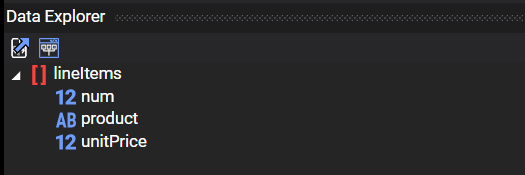# Dynamic Color Using Designer

You can dynamically change the color of fields using expressions.

DynamicPDF Cloud API now allows conditionally formatting an element's color using expressions. In the following example we illustrate formatting a simple DLEX Report.

infoAfter opening the `DynamicColorTest.dlex` file in Designer you see five different formatted elements, each formatted using an expression.The following table summarizes the elements and the expressions used.

ElementExpressionExplanation
ASymbol`IF(LTE(num,0),"red",LT(num,10),"yellow")`If `num <= 0` then red checkmark. Else if `num <=10` then yellow checkmark. Otherwise, no checkmark (as default is omitted).
BLine`IF(LTE(num,0),"red",LT(num,10),"yellow", "purple")`If `num <= 0` then red line. Else if `num <=10` then yellow line. Otherwise, purple line (the default).
CRecordBox`IF(GTE(num,10), "green","black")`If `num >= 10` then green text otherwise black.
DRecordBox`IF(LTE(num,0),"critical")`If `num <= 0` then display `critical` otherwise do not display.
ERectangle`IF(GT(num,10),"blue",LTE(num,0),"red","green")`If `num > 10` then blue. Else if `num <= 0` then red. Otherwise green (default).

The Data Explorer pane contains the dataset, consisting of `lineItems` with a `num`, `product`, and `unitPrice` field.The Layout Data Editor contains the complete JSON document.## Formatting Symbol​

The checkmark uses a Symbol element to display a `symbolType` of `check1`. However, if the color of the checkmark is either red, yellow, or omitted depending upon the value of the `num` field.

`IF(LTE(num,0),"red",LT(num,10),"yellow")`## Formating Recordbox​

The RecordBox containing the `num` field is either green or black, depending upon its value.

`IF(GTE(num,10), "green","black")`## Formatting Line​

The Line is either red, yellow, or purple depending upon the value of the `num` field.

`IF(LTE(num,0),"red",LT(num,10),"yellow", "purple")`## Formatting Rectangle​

The Rectangle element's color is blue, red, or green depending upon the `num` field's value.

`IF(GT(num,10),"blue",LTE(num,0),"red","green")`## Generated Report​

Running the report generates the following PDF.## DLEX Document​

``<document xmlns="https://www.dynamicpdf.com" xmlns:xsi="http://www.w3.org/2001/XMLSchema-instance" xsi:schemaLocation="https://www.dynamicpdf.com https://www.dynamicpdf.com/schemas/DLEX20.xsd" version="2.0" author="" keywords="" subject="" title="" id="Document1">  <report id="Report1" dataName="lineItems" pageSize="letter" pageOrientation="portrait" leftMargin="50" topMargin="50" rightMargin="50" bottomMargin="50">    <template id="Template1"/>    <header id="Header1" height="20">      <label id="Label2" x="3" y="4" width="69" height="14" underline="false" text="PRODUCT"/>      <label id="Label3" x="76" y="4" width="100" height="14" align="right" underline="false" text="UNIT PRICE"/>      <label id="Label4" x="181" y="4" width="100" height="14" align="right" underline="false" text="CREDITS"/>      <line id="Line1" x1="2" y1="20" x2="512" y2="20"/>    </header>    <detail id="Detail1" autoSplit="false" height="18">      <symbol id="Symbol1" x="283" y="3" width="43" height="14" color="IF(LTE(num,0),"red",LT(num,10),"yellow")" symbolType="check1"/>      <recordBox id="RecordBox2" x="3" y="3" width="68" height="14" underline="false" autoLeading="true" cleanParagraphBreaks="true" expandable="false" rightToLeft="false" splittable="false" dataName="product"/>      <recordBox id="RecordBox4" x="179" y="3" width="100" height="14" align="right" textColor="IF(GTE(num,10),"green","black")" underline="false" autoLeading="true" cleanParagraphBreaks="true" expandable="false" rightToLeft="false" splittable="false" dataName="num"/>      <recordBox id="RecordBox5" x="76" y="3" width="100" height="14" align="right" underline="false" autoLeading="true" cleanParagraphBreaks="true" expandable="false" rightToLeft="false" splittable="false" dataName="unitPrice" dataFormat="\$#.00"/>      <rectangle id="Rectangle1" x="476" y="3" width="30" height="14" borderColor="IF(GT(num,10),"blue",LTE(num,0),"red","green")"/>      <recordBox id="RecordBox7" x="330" y="3" width="66" height="14" align="center" textColor="red" underline="false" autoLeading="true" cleanParagraphBreaks="true" expandable="false" rightToLeft="false" splittable="false" dataName="IF(LTE(num,0),"critical")"/>      <line id="Line2" x1="401" y1="10" x2="457" y2="10" color="IF(LTE(num,0),"red",LT(num,10),"yellow","purple")"/>    </detail>    <footer id="Footer1" height="20">      <conditionalFooter id="ConditionalFooter1" condition="LastPage">        <recordBox id="RecordBox6" x="410" y="3" width="100" height="14" align="right" underline="false" autoLeading="true" cleanParagraphBreaks="true" expandable="false" rightToLeft="false" splittable="false" dataName="sum(num)"/>        <label id="Label5" x="306" y="3" width="100" height="14" underline="false" text="TOTAL CREDITS:"/>      </conditionalFooter>    </footer>  </report></document>``